# AP Physics C Electricity : Using Gauss's Law

## Example Questions

### Example Question #12 : Electricity

A charge,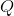, is enclosed by two spherical surfaces of radii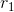and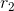, with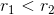. The cross-sectional side view is shown.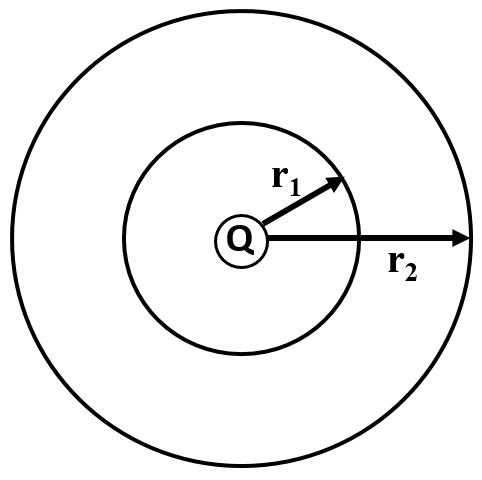Which is the correct relationship between the electric flux passing through the two spherical surfaces around the point charge?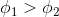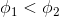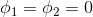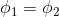Explanation:

Electric flux is given by either side of the equation of Gauss's Law: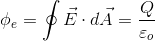Since the charge is the same for both spherical surfaces, even though these surfaces are of different radii, the amounts of electric flux passing through each surface is the same.

### All AP Physics C Electricity Resources